# Quick demonstration of GNU Octave¶

In a Jupyter Notebook

## Some basic plotting¶

Let show some basic use of GNU Octave for computations, and plotting.

In :
% Some variables
N = 100
disp(['Number of values N =', num2str(N)])
h = 1 / N
disp(['Step h = ', num2str(h)])

N =  100
Number of values N =100
h =  0.010000
Step h = 0.01

In :
% Some arrays
t = 0 : h : 2*pi;
x = cos(t);
y = sin(t);
length(t)
length(x)
length(y)

ans =  629
ans =  629
ans =  629

In :
fig = figure();
hold on
grid on
plot(t, x, 'r*-')
plot(t, y, 'b+-')
legend('cos(t)', 'sin(t)')
title('Cosinus and sinus on [0, 2 \pi]')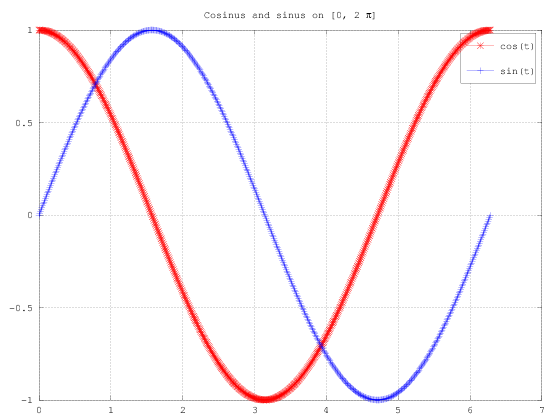In :
fig = figure();
plot(x, y);
title('sin(t) as function of cos(t)')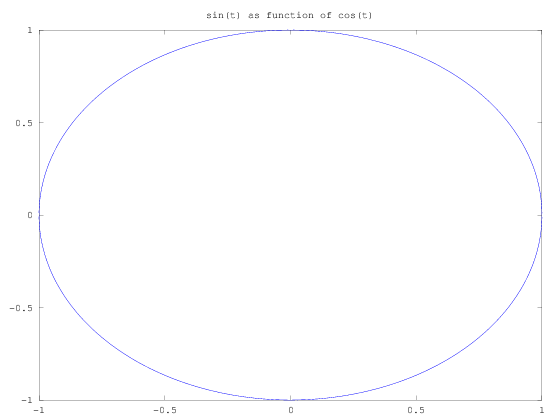## Some basic linear algebra¶

We can also easily work with matrices and showcase a little bit of linear algebra.

In :
a = [[1 0 1]; [0 1 1]; [1 1 0]]

a =

1   0   1
0   1   1
1   1   0


In :
a'  # a transpose

ans =

1   0   1
0   1   1
1   1   0


In :
b = 1 + a^5

b =

12   11   12
11   12   12
12   12   11



eig gives the eigen values:

Test of notes.

In :
l_a = eig(a)
l_b = eig(b)

l_a =

-1.00000
1.00000
2.00000

l_b =

-1.00000
1.00000
35.00000


In :
[Ua, Sa, Va] = svd(a)

Ua =

-5.7735e-01   4.0825e-01  -7.0711e-01
-5.7735e-01  -8.1650e-01  -7.8505e-17
-5.7735e-01   4.0825e-01   7.0711e-01

Sa =

Diagonal Matrix

2.00000         0         0
0   1.00000         0
0         0   1.00000

Va =

-0.57735   0.81650  -0.00000
-0.57735  -0.40825   0.70711
-0.57735  -0.40825  -0.70711


In :
[Ub, Sb, Vb] = svd(b)

Ub =

-5.7735e-01   4.0825e-01  -7.0711e-01
-5.7735e-01  -8.1650e-01  -1.6098e-15
-5.7735e-01   4.0825e-01   7.0711e-01

Sb =

Diagonal Matrix

35.00000          0          0
0    1.00000          0
0          0    1.00000

Vb =

-0.57735   0.81650  -0.00000
-0.57735  -0.40825   0.70711
-0.57735  -0.40825  -0.70711



## 3D plotting¶

Some basic 3D plotting:

In :
x = linspace(-2, 2, 50);
y = linspace(-2, 2, 50);
[xx,yy] = meshgrid(x, y);
mesh(xx, yy, 4 - (xx.^2 + yy.^2))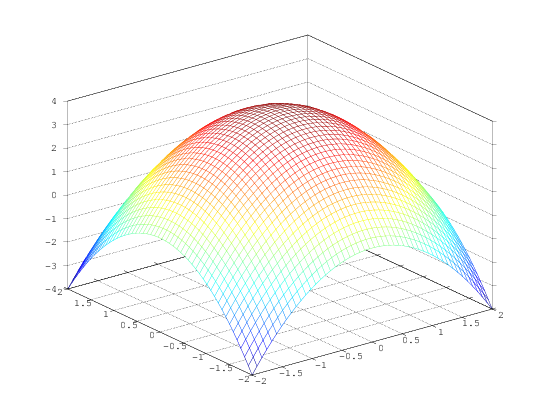In :
x = linspace(-2, 2, 50);
y = linspace(-2, 2, 50);
[xx,yy] = meshgrid(x, y);
meshc(xx, yy, 4 - (xx.^2 + yy.^2))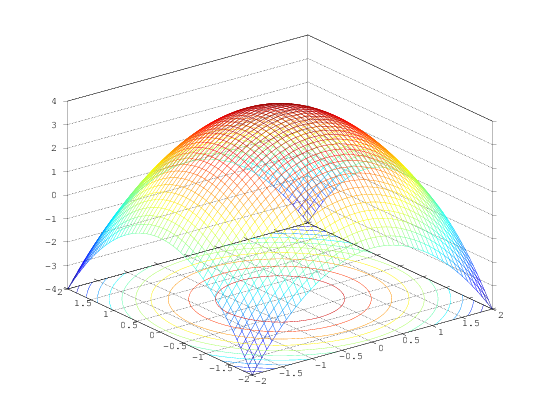In :
# From https://octave.sourceforge.io/octave/function/plot3.html
z = [0:0.05:5];
plot3(cos (2*pi*z), sin (2*pi*z), z, ";helix;");
plot3(z, exp (2i*pi*z), ";complex sinusoid;");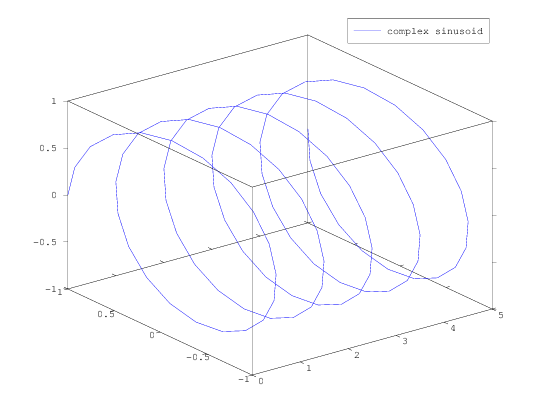In :
clf;
z = [0:0.05:5];
plot3 (cos (2*pi*z), sin (2*pi*z), z);
legend ("helix");
title ("plot3() of a helix");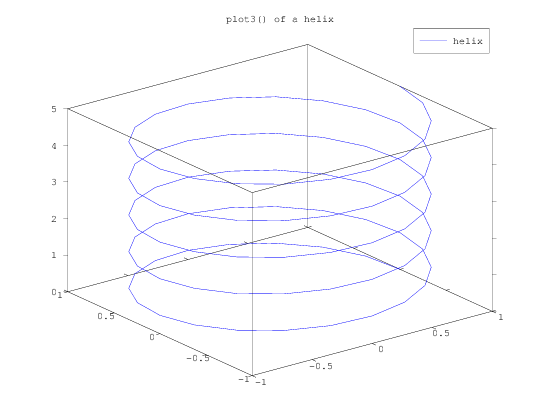In :
clf;
colormap ("default");
[X, Y] = meshgrid (linspace (-3, 3, 40));
Z = sqrt (abs (X .* Y)) ./ (1 + X.^2 + Y.^2);
meshc (X, Y, Z);
title ("meshc() combines mesh/contour plots");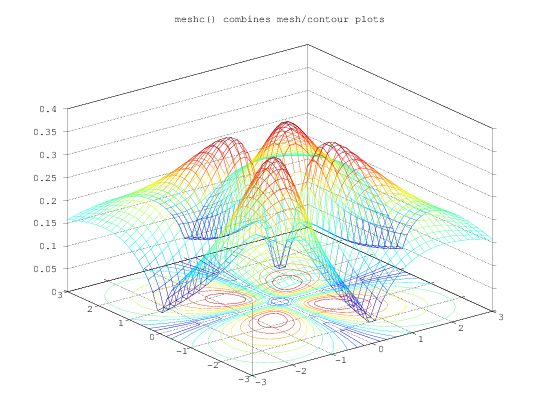## Conclusion¶

We showed a little bit how to use a Jupyter notebook with GNU Octave (or GNU Octave with a Jupyter notebook?).

That's all for today, folks!